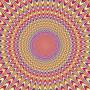# Hard Brain Teasing Quiz!There are many people who can control their brain more than other people do. They, usually, learns how to do so by taking English riddles, Mathematical riddles and other kinds of riddles. Some of those riddles are collected here.

Ready to challenge your brain? Take this riddles to find out, whether you are like those persons or not. This consists of Mathematical questions the most. Good luck!

Created by: Jeeshan

1. My grandson is about as many days as my son in weeks, and my grandson is as many months as I am in years. My grandson, my son and I together are 120 years. Can you tell me my age in years ?
2. Given these equations, what does 4500 equal? 8898=7 4566=2 1203=1 2313=0 4566=2 5464=1 7774=0 1003=2 9856=4 9955=2 1886=5 1231=0 8764=3 4500=?
3. How many times can you subtract the number two from the number fifty?
4. What number comes next in the following sequence? 2 4 8 10 20 _
5. What letter comes next in the following sequence? D R M F S L T_
6. If you wrote all of the numbers from 300 to 400 on a piece of paper, how many times would you have written the number 3?
7. Find a number less than 100 that is increased by one-fifth of its value when its digits are reversed.
8. 2+3=8, 3+7=27, 4+5=32, 5+8=60, 6+7=72, 7+8=??
9. At a party, everyone shook hands with everybody else. There were 66 handshakes. How many people were at the party?
10. The value of x + x(x^x) when x = 2 is:

Remember to rate this quiz on the next page!
Rating helps us to know which quizzes are good and which are bad.

What is GotoQuiz? A better kind of quiz site: no pop-ups, no registration requirements, just high-quality quizzes that you can create and share on your social network. Have a look around and see what we're about.